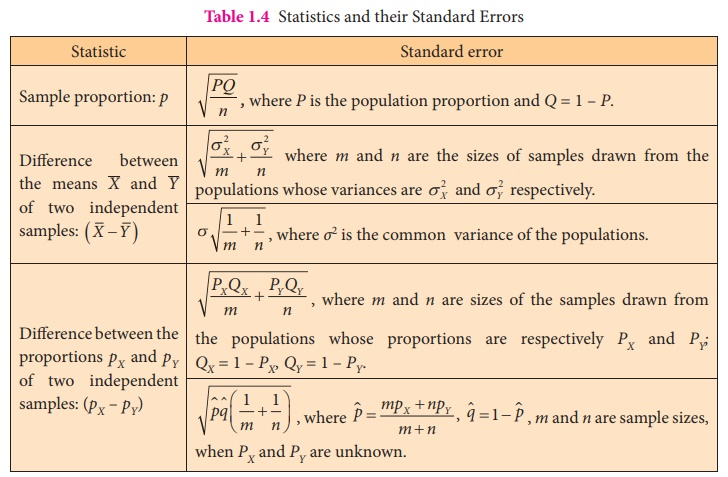Home | | Statistics 12th Std | Standard Error

# Standard Error

The standard deviation of the sampling distribution of a statistic is defined as the standard error of the statistic, which is abbreviated as SE.

STANDARD ERROR

The standard deviation of the sampling distribution of a statistic is defined as the standard error of the statistic, which is abbreviated as SE.

For example, the standard deviation of the sampling distribution of the sample mean, x, is known as the standard error of the sample mean, or SE ().

If the random variables X1, X2, ..., X–n are independent and have the same distribution with mean μ and variance σ2, then variance ofbecomes as## Example 1.2

Calculate the standard error offor the sampling distribution obtained in Example 1.

### Solution:

Here, the population is {4, 8, 12, 16}.

Population size (N) = 4, Sample size (n) = 2

Population mean (µ) = (4 + 8 + 12+ 16)/4 = 40/4 = 10

The population variance is calculated asThis can also be verified from the sampling distribution of(see Table 1.3)Hence, the standard deviation of the sampling distribution ofis = √10 .

Standard Errors of some of the frequently referred statistics are listed in Table 1.4.

Table 1.4 Statistics and their Standard ErrorsTags : Definition, Example Solved Problems | Statistics , 12th Statistics : Chapter 1 : Tests of Significance - Basic Concepts and Large Sample Tests
Study Material, Lecturing Notes, Assignment, Reference, Wiki description explanation, brief detail
12th Statistics : Chapter 1 : Tests of Significance - Basic Concepts and Large Sample Tests : Standard Error | Definition, Example Solved Problems | Statistics